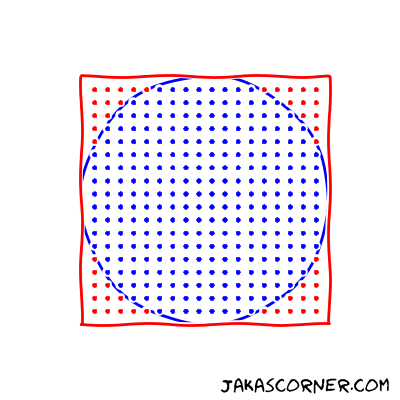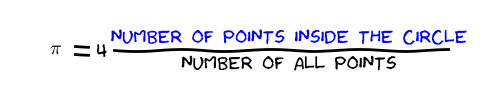In the previous article we studied a sequential Monte Carlo algorithm for approximating Pi (`π`).

In this article we briefly repeat the idea of the sequential algorithm. Then, we parallelize the sequential version with the help of OpenMP.

## The sequential algorithm

A Monte Carlo algorithm for approximating `π` uniformly generates the points in the square ```[-1, 1] x [-1 1]```. Then it counts the points which lie in the inside of the unit circle.An approximation of `π` is then computed by the following formula:For more details about the formula look in the previous article.

The source code for the approximation algorithm is

It uses `UniformDistribution` to generate samples in `[-1, 1]`. Then it counts the number of points in the inside of the circle and computes an approximation of `π` by the approximation formula.

## Parallelization with OpenMP

The task is to parallelize the approximation algorithm with the help of OpenMP.

Looking at the function `approximatePi`, we might be tempted to simply parallelize the for loop in the function with ```#pragma omp parallel for```. But unfortunately, this approach has some problems. It introduces data races in the program.

The reasons for the data races are the variables `distribution` and `counter`. They are updated by different iterations. If we would parallelize the for loop, then the variables might be updated by different threads at the same time.

Note that the `distribution` is updated in every iteration, because the sampling updates the internal state of the procedure which generates the random samples.

Since a simple parallelization of the `approximatePi` is not possible, we slightly change the existing code.

# Modifications

Our strategy is to divide the sampling into chunks. This strategy introduces two nested loops

• an outer loop over the chunks and
• an inner loop over the samples for each chunk.

The code for the outer loop is presented below

The function `parallelPi` divides the number of total samples into eight chunks. The `chunk` variable holds the number of samples for each chunk. Then the function loops over each chunk. In the for loop, the function `samplesInsideCircle` generates samples and counts the number of samples in the inside of the unit circle. In the end, the `parallelPi` computes an approximation of the `π` with the help of the known formula.

The missing piece is the function `samplesInsideCircle`. It is presented below.

This function is very similar to the `approximatePi`. The `samplesInsideCircle` generates `numSamples` uniform samples and counts how many of them are in the inside of the circle.

# Parallelization

Now, we are ready for a parallelization of the algorithm. We choose to parallelize the for loop over the chunks, since it is the outer for loop.

In this for loop

we have three shared variables: `numChunks`, `counter` and `chunk`. We do not modify the `numChunks` and `chunk`. They are read-only variables. Therefore, this is not a problem. On the other hand, we modify the `counter`. We must deal with the problem of data races for this variable.

Before we continue, let us remember the sequential version of the program. There we have problems (data races) with two variables: `distribution` and `counter`. The `counter` is still problematic but we do not have a problem with the `distribution`. We avoided this problem by having a different `distribution` for each chunk. With other words, the sequential version initializes one object of `UniformDistribution` and the parallel version initializes `numChunks` objects of `UniformDistribution`.

Now, we continue with the problem of `counter`.

OpenMP has a special clause of the loop construct which solves this kind of problems. This is the `reduction` clause which can specify a reduction. An example of a reduction is

Here, `+` is the operation which reduces `a0`, `a1`, …, `a9` into the `sum`.

The reduction clause requires two things to specify a reduction

• the reduction operation and
• the variable, which will hold the result of the reduction.

In the for loop, `counter` is the variable, which will hold the result and `+` is the reduction operation. The loop construct then looks like

This loop construct correctly parallelizes the for loop. OpenMP increments the shared variable `counter` without introducing a data race.

## Example

We run the sequential and parallel versions of the Monte Carlo algorithm for approximating `π`. We measure the execution time of the both versions. The source code is available here.

We can see that the execution time of the parallel version (3.6 seconds) is lower than the execution time of the sequential version (20 seconds). Both results are accurate up to four decimal places. I ran the computations on my machine which has 4 two-threaded cores.

In the next article, we will look in more detail at the reduction clause.

## Summary

We parallelized a Monte Carlo method for approximating `π`. We saw that the parallelization can decrease the execution time.